Function Repository Resource:

# AssociationNormalize

Normalizes the values of an Association

Contributed by: Seth J. Chandler
 ResourceFunction["AssociationNormalize"][assoc] divides each value in assoc by the Norm of its values. ResourceFunction["AssociationNormalize"][assoc,f] divides each value in assoc by a function f of its values.

## Details

Consistent with the way Normalize behaves, if all the values in an association are zero, ResourceFunction["AssociationNormalize"] returns its first argument unchanged.
ResourceFunction["AssociationNormalize"] evaluates all values in assoc, including those stored with RuleDelayed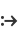.

## Examples

### Basic Examples (2)

Normalize an Association with three values:

 In:=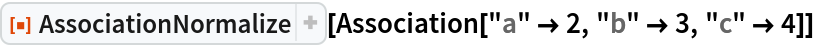Out=Normalize an Association with three values so that the values total to 1:

 In:=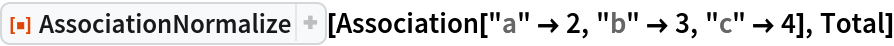Out=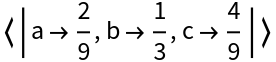### Scope (4)

Symbolic values:

 In:=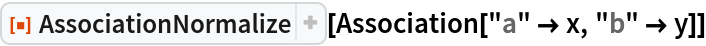Out=Use an arbitrary norm function:

 In:=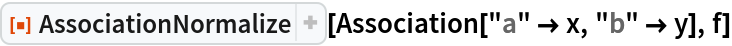Out=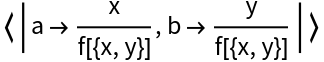An association with complex values:

 In:=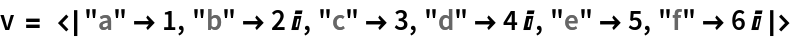Out=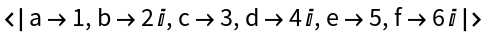Normalize using exact arithmetic:

 In:=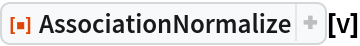Out=An association where all the values are zero:

 In:=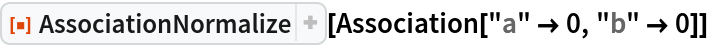Out=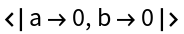### Applications (4)

Here is an association in which the keys are various attributes and the values are probabilities (the problem is taken from the Probabilistic Graphical Models book contained in the Sources section). The goal is to figure out the probability that the value of "sat" is "s1" given that the grade is "s2":

 In:=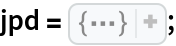Eliminate any associations in which the value of "grade" is not "g2":

 In:=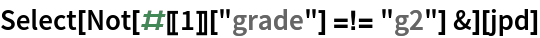Out=Normalize the remaining probabilities:

 In:=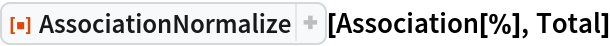Out=Use KeySelect to compute the probability that the "sat" value is "s1":

 In:=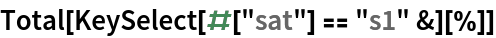Out=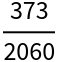### Possible Issues (1)

Delayed values are evaluated:

 In:=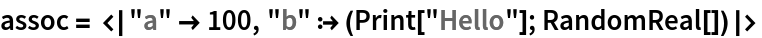Out=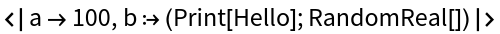In:=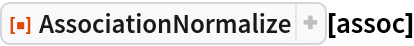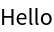Out=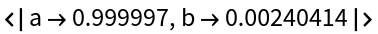Seth J. Chandler

## Version History

• 1.0.0 – 06 July 2021